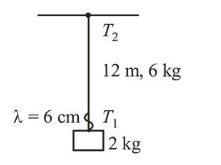# A uniform thin rope of length 12m and mass 6kg hangs vertically`
Question:

A uniform thin rope of length $12 \mathrm{~m}$ and mass $6 \mathrm{~kg}$ hangs vertically from a rigid support and a block of mass $2 \mathrm{~kg}$ is attached to its free end. A transverse short wave-train of wavelength $6 \mathrm{~cm}$ is produced at the lower end of the rope. What is the wavelength of the wavetrain (in $\mathrm{cm}$ ) when it reaches the top of the rope?

1. 3

2. 6

3. 12

4. 9

Correct Option: , 3

Solution:

(3) Using, $V=f \lambda$

$\frac{V_{1}}{\lambda_{1}}=\frac{V_{2}}{\lambda_{2}} \Rightarrow \lambda_{2}=\frac{V_{2}}{V_{1}} \lambda_{1}$Again using,

$n=\frac{V}{\lambda}=\sqrt{\frac{T}{M}} \lambda_{2}=\sqrt{\frac{T_{2}}{T_{1}} \lambda_{1}} \quad T_{2}=8 \mathrm{~g}($ Top $)$

$=\sqrt{\frac{8 g}{2 g}} \lambda_{1}=2 \lambda_{1}=12 \mathrm{~cm} \quad T_{1}=2 \mathrm{~g}$ (Bottom)